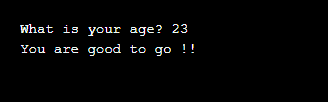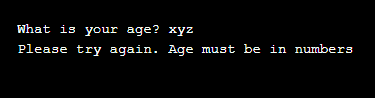CppBuzz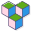# Python Cheat Sheet for Beginners

Cheat sheet covers print, concatenation, list, tuples, if-elif-else statements, dictionaries, user input, while loops, classes, files I/O, functions, exceptions etc..

## Printing "Hello World" in Python

`print("Hello World");`

## Variable in Python

```variable = "Hello World!"
print(variable)```

## Concatenation of Strings

```FirstName = 'Cpp'
LastName = 'Buzz'
FullName = FirstName + ' ' + LastName
print(FullName)
```

## Taking User Input in Python

Accepting String values

```name = input("What's your name? ")
print("Hello, " + name + "!")
```

Accepting Integer values

```age = input("How old are you? ")
age = int(age)
cm = input("C.M in on Inch? ")
cm = float(cm)
```

## Examples on Lists

list is a series of items. Lists are accessed using index, or loops.

Make a list
fruits = ['apple', 'banana', 'orange']

Getting the first element from list
FirstFruit = fruits

Getting the last element from list
LastFruit = fruits[-1]

Looping through list
for fruit in fruits: print(fruit)

Appending Elements to list
fruits.append('pineapple') fruits.append('grapes')

## Tuples

Tuples are similar to lists. With only one difference: a tuple can't be modified. Tuples are immutable

Making a tuple

```AgeGroup = (1,2,3,4,5)
print (AgeGroup)
print (AgeGroup[-1])
print (AgeGroup)
```

Output of above python code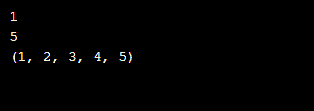## If elif & else statements in Python

```age = input("How old are you? ")
age = int(age)

if(age<18):
print("You are less than 18")

elif (age>=18 and age<=60):
print("You are older than 18 but younger than 60")
else:
print("You are older than 60")
```
Output of above Python code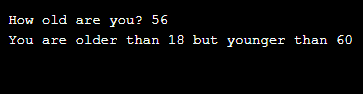## Dictionaries

Dictionary stores elements in key-value pair. Each item in a dictionary is a key-value pair.

Example of Dictionary

```Avg_American =  {'color': 'white', 'height': '5.10'}
print("\nAmerican's color is : " + Avg_American['color'])

print('\n')

for key, value in Avg_American.items():
print('Key : ' + key + ' ,with Value : ' + value)

#Looping through all keys
print('\n')
for color in Avg_American.keys():
print(color + ' is one key \n')

#Looping through all the values

for height in Avg_American.values():
print(height + ' is one value \n')
```

Output of Dictionary code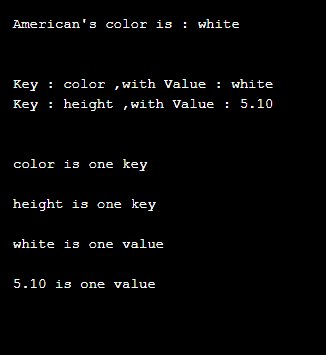## While Loop

```var = 1
while var <= 5:
print(var)
var += 1
```

Output of above while loop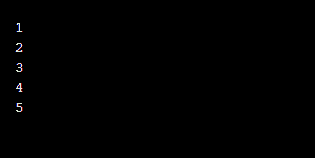## For loop

```for a in range(1, 6):
print(a)
```

Output of above for loop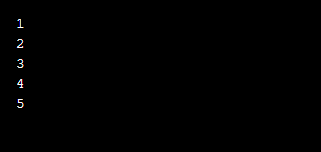Looping through String

```for x in "cppbuzz":
print(x)
```

Output of above for loop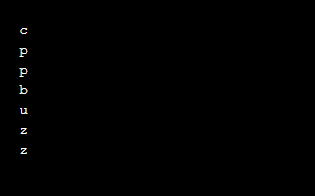## Functions

Function is a group of Python statements, designed to perform specific job. Functions can also accept arguments

```#this is function definition
def myFunction():
print("Welcome to CppBuzz !!")

#this is function call
myFunction()
```Passing argument to functions

```#this is function definition
def myFunction(arg):
print(arg + " Welcome to CppBuzz !!")

#this is function call
myFunction('Dear Users')```

Output of above Python code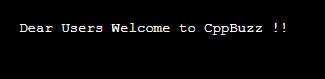Returning value from function

```def add_num(x, y):
#Add two numbers and returning their sum
return x + y

print(sum) ```## Class

Class defines the behavior of any object and kind of information an object can store.

```class CppBuzz():
def __init__(self, type):
"""Initialize dog object."""
self.type = type

def open(self):
"""Simulate Opening."""
print(self.type + " CppBuzz is opening.")

def close(self):
"""Simulate Closing."""
print(self.type + " CppBuzz is closing.")

obj = CppBuzz('Website')
print("Cppbuzz is a : " + obj.type)
obj.open()
obj.close();```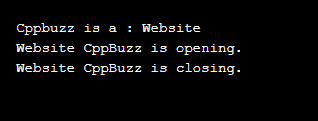## Files I/O in Python

Files are used to store the information permanently. In Python open() function is used to open file, we can also pass different arguments to this function like 'r', 'w'. By default 'r' mode is chosen while opening any file.

```filename = 'cppbuzz.txt'
with open(filename, 'a') as file_object:
file_object.write("\nI love CppBuzz.")

#Reading a file and storing its lines
filename = 'cppbuzz.txt'
with open(filename) as file_object:

for line in lines:
print(line)

#Writing to a file
filename = 'cppbuzz.txt'
with open(filename, 'w') as file_object:
file_object.write("CppBuzz - a portal to improve programming skills.")

#Appending to a file
filename = 'cppbuzz.txt'
with open(filename, 'a') as file_object:
file_object.write("\nI love CppBuzz.")

filename = 'cppbuzz.txt'
with open(filename) as file_object:

for line in lines:
print(line)
```

Output of above Python code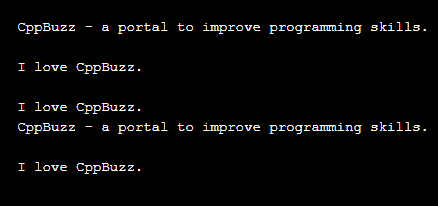## Exceptions

Exceptions are run time error, which my occur due to any wrong condition. Catching exception help us in showing appropriate error to end user.

```prompt = "How many tickets do you need? "
num_tickets = input(prompt)
try:
num_tickets = int(num_tickets)
except ValueError: